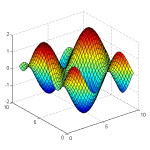# Calculus courses

Learn calculus with the following free courses and tutorials. Chosen and upvoted by our community. Do you know other free Calculus courses that should be listed here? Add them to Coursearena and help others who search for it.

### Free Calculus courses and tutorialsExplore advanced precalculus topics and gain the mathematical knowledge and skills required to take a first course in precalculus.AP® Calculus BC[www.edx.org]
Learn AP® Calculus BC topics from highly respected AP Calculus instructors to prepare for the AP® Calculus BC exam.Master the most difficult topics in your AP Calculus AB & Calculus BC courses.Get an introduction to the essential mathematical knowledge and skills required to take a first course in calculus.Prepare for Introductory Calculus courses.Calculus Applied![www.edx.org]
Apply tools of single-variable calculus to create and analyze mathematical models used by real practitioners in social, life, and physical sciences.Precalculus[www.edx.org]
This college-level, Precalculus course will teach you the skills required for success in future Calculus studies.

### Calculus Tips & Tricks from our community

We don't have any tips and tricks for this topic yet. Do you have any? We would love to hear about it. Sign up!
Course type
Difficulty level
Costs

### Similar topics that might be interesting for you##### Mathematics
4 courses available
Science/Mathematics##### Math puzzles
2 courses available
Science/Mathematics##### High School Math
3 courses available
Science/Mathematics##### Computational Mathematics
5 courses available
Science/Mathematics